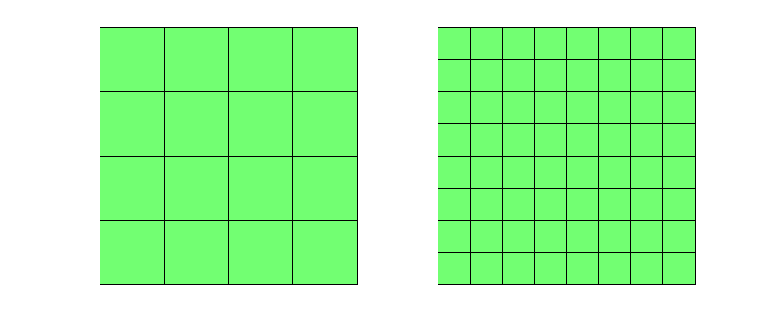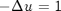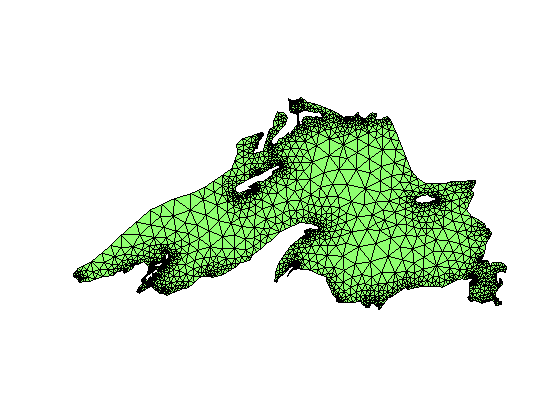# Project: Multigrid Methods

In this project we will learn three ways of implementating multigrid methods: from matrix-free version to matrix-only version depending on how much information on the grid and PDE is provided.

## Multigrid on Uniform Grids for Poisson Equations

We consider linear finite element or equivalently 5-point stencil discretization of the Poisson equation on a uniform grid of [0,1]^2 with size h. For simplicity, we assume h = 1/2^L and zero Dirichlet bounary condition.

```set(gcf,'Units','normal'); set(gcf,'Position',[0,0,0.4,0.3]);
subplot(1,2,1); showmesh(node,elem);
subplot(1,2,2); showmesh(node,elem);
```## Step 1 Smoother

• Check the convergence of Gauss-Seidel smoother by solvingin (0,1)^2 with zero Dirichlet boundary condition. Plot the error in suitable norm vs iteration for h = 1/64.
• Change h from 1/4 to 1/128 and compare the iterations to drive the relative error in a suitable norm below the discretiztion error h^2.
• Choose a random initial guess. Plot the error function on the grid for the first 3 steps.
• (Optional) Code the red-black Gauss-Seidel.

## Step 2 Two-Grid method

• Figure out the index map between fine grid with size h and coarse grid with size 2h. For example, (i,j) in coarse grid is (2*i-1,2*j-1) in the fine grid.
• Code the bilinear prolongation and restriction using the index map. Be carefuly on the value on the boundary points.
• Code the two-grid method. On the fine grid, apply m times G-S iteration and then restrict the updated residual to the coarse grid. On the coarse grid, use G-S iteration or direct method to solve the equation below the discretization error. Then prolongates the correction to the fine grid and apply additional m G-S iterations.
• Use the two-grid method as an iteration to solve the Poisson equation.
• Change h from 1/4 to 1/128 and compare the iterations of two-grid methods for different h.

## Step 3 V-cycle Multi-grid method

`Choose one of the following approach to implement the MG.`
• (Recrusive way) Apply the two-grid method to the coarse grid problem in Step 2.
• (Non-recrusive way) Follow the description of SSC in lecture notes to implement V-cycle.
• Use the Vcycle as an iteration to solve the Poisson equation, i.e.,
```u = u + Vcycle(r,J); % correction form
u = Vcycle(u,J); % direct update form```
• Change h from 1/4 to 1/128 and check the iterations and cpu time of MG.

## Multigrid on Hierarchical Grids

We consider linear finite element discretization of the Poisson equation on grids obtained by uniform refinement of a coarse grid.

```[node,elem] = circlemesh(0,0,1,0.25);
subplot(1,2,1); showmesh(node,elem);
[node,elem] = uniformrefine(node,elem);
subplot(1,2,2); showmesh(node,elem);
```
``` - Min quality 0.8507 - Mean quality 0.9612 - Uniformity 3.93%
```## Step 1 Hierarchical Meshes

Generate the initial grid by

```[node,elem] = circlemesh(0,0,1,0.25);
```
``` - Min quality 0.8507 - Mean quality 0.9612 - Uniformity 3.93%
```
• Refine the initial mesh J times to get the finest mesh. To get a mesh of the disk, the boundary nodes should be projected onto the unit circle.
• Construct HB in two ways. Either from the output of uniformrefine during the refinement or call uniformcoarsenred from the finest mesh.

## Step 2 Transfer Operator and Smoothers

• Assemble the stiffness matrix A in the finest mesh.
• Construct prolongation and restriction matrices using HB.
• Compute stiffness matrix in each level by the triple product.
• Store the smoother tril(A) and triu(A) in each level.

## Step 3 V-cycle Multigrid

Be careful on the boundary nodes. Restrict smoothing to interiori nodes only and enforce the residual on boundary nodes to be zero.

• For one single grid, say J = 4, show the decrease of the residual in certain norm for each iteration of the multigrid method.
• Test V-cycle MG for J = 3:6. List iteration steps and cpu time.

## Algebraic Multigrid Method

We consider solving an SPD matrix equation Ax = b, where A could be obtained as the finite element discretization on a unstructured grids. A coarsening of the graph of A is needed and restriction and prolongation can be constructued based on the coarsening.

## Step 1 Matrix

• Load the lakemesh.mat in ifem/data.
• Assemble the stiffness matrix on this mesh and take the submatrix associated to interior nodes only.

The mesh is only used to generate the matrix. In the later step, only the generated matrix is used.

```load lakemesh.mat;
figure; clf; showmesh(node,elem);
A = assemblematrix(node,elem);
```## Step 2 Coarsening

Use coarsenAMGc to get a set of coarse nodes.

```help coarsenAMGc
```
```  COARSENAMGC coarsen the graph of A.

isC = coarsenAMGc(A) terturns a logical array to make a set of nodes as
the coarse ndoes based on As, a strong connectness matrix modified from
A. The strong connectness is slightly different with the standard
definition.

[isC,As] = coarsenAMGc(A,theta) accepts the parameter theta to define the
strong connectness. The default setting is theta = 0.025. It also outputs
the strong connectness matrix As which could be used in the constrction
of prolongation and restriction.

Example
A = assemblematrix(node,elem);
[isC,As] = coarsenAMGc(A);

Reference page in Help browser
<a href="matlab:ifem coarseAMGdoc">coarsenAMGdoc</a>

```

## Step 3 Transfer Operator and Smoothers

Use interpolationAMGs to get restriction and prolongation operators.

```help interpolationAMGs
```
```  INTERPOLATIONAMGS construct prolongation and restriction matrices

[Pro,Res] = interpolatoinAMGs(A,isC) construct prolongation and
restriction matrices use standard matrix-dependent interpolation.

In the input, A is a SPD matrix and isC is a logical array to indicate
nodes in coarse matrix. In the output Pro and Res are prolongation and
restriction matrices satisfying Res = Pro'.

The submatrix A_{cf} is used to construct the interpolation of values on
fine nodes from that of coarse nodes. The weight is normalized to
preserve the constant.

Example
A = assemblematrix(node,elem);
[isC,As] = coarsenAMGc(A);
[Pro,Res] = interpolationAMGs(As,isC);